Anúncio

30 de Sep de 2015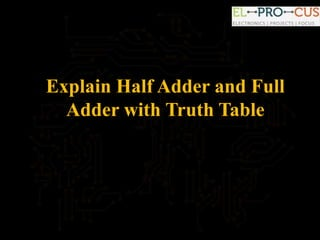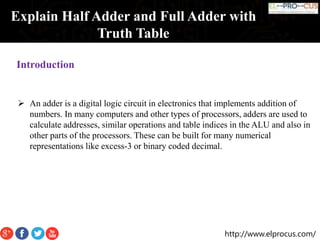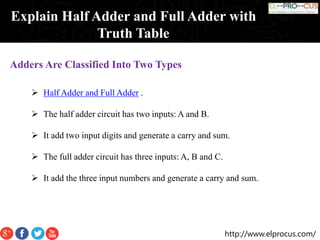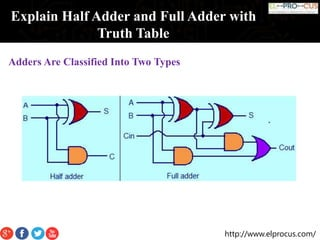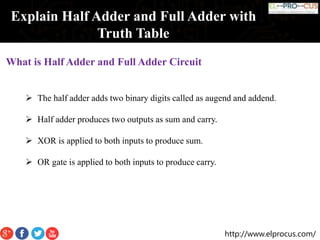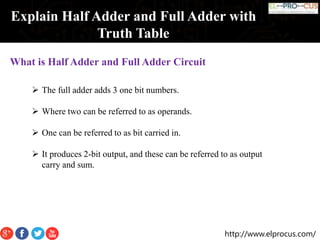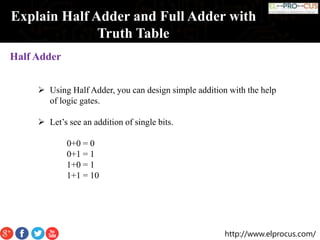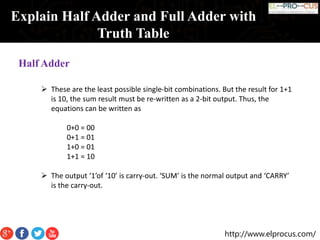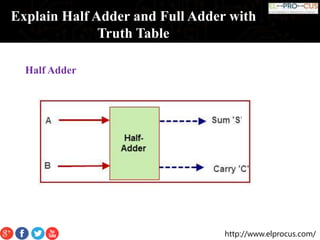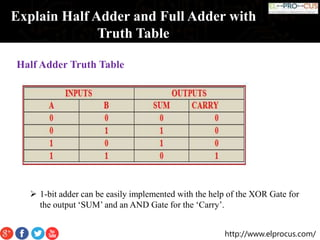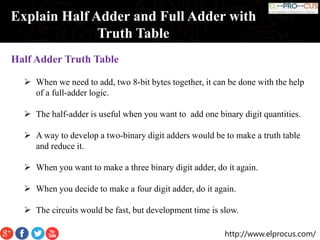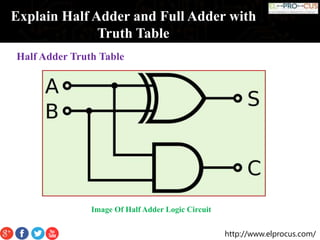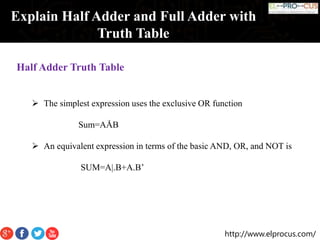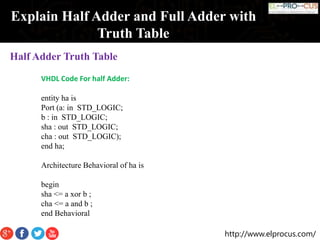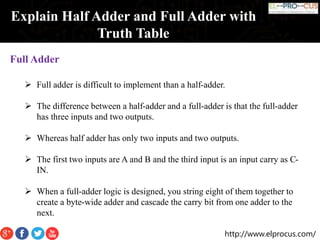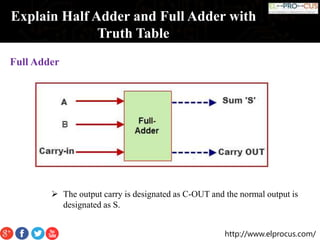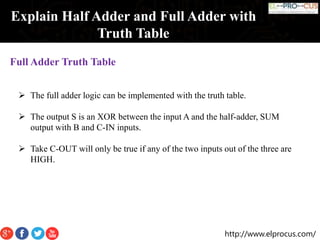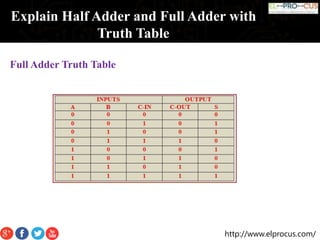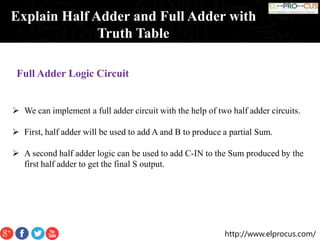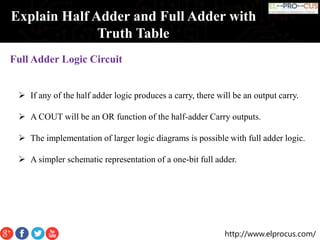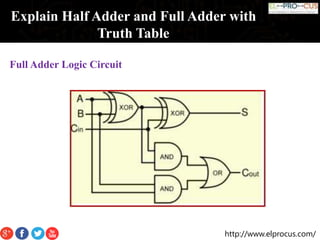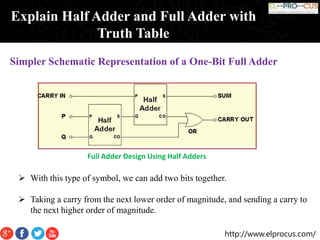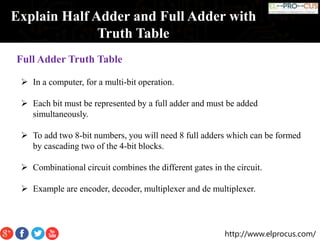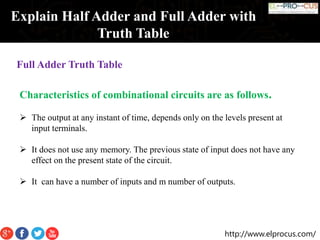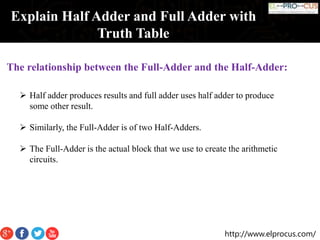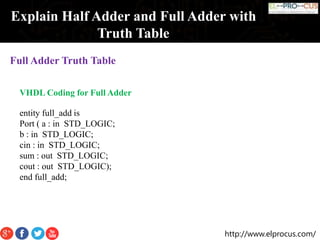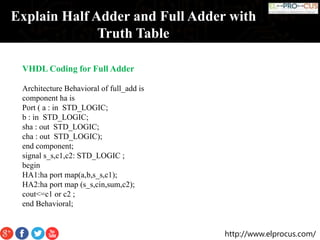1 de 28
Anúncio

2. http://www.elprocus.com/ Explain Half Adder and Full Adder with Truth Table  An adder is a digital logic circuit in electronics that implements addition of numbers. In many computers and other types of processors, adders are used to calculate addresses, similar operations and table indices in the ALU and also in other parts of the processors. These can be built for many numerical representations like excess-3 or binary coded decimal. Introduction
3. http://www.elprocus.com/ Explain Half Adder and Full Adder with Truth Table Adders Are Classified Into Two Types  Half Adder and Full Adder .  The half adder circuit has two inputs: A and B.  It add two input digits and generate a carry and sum.  The full adder circuit has three inputs: A, B and C.  It add the three input numbers and generate a carry and sum.
4. http://www.elprocus.com/ Explain Half Adder and Full Adder with Truth Table Adders Are Classified Into Two Types
5. http://www.elprocus.com/ Explain Half Adder and Full Adder with Truth Table What is Half Adder and Full Adder Circuit  The half adder adds two binary digits called as augend and addend.  Half adder produces two outputs as sum and carry.  XOR is applied to both inputs to produce sum.  OR gate is applied to both inputs to produce carry.
6. http://www.elprocus.com/ Explain Half Adder and Full Adder with Truth Table What is Half Adder and Full Adder Circuit  The full adder adds 3 one bit numbers.  Where two can be referred to as operands.  One can be referred to as bit carried in.  It produces 2-bit output, and these can be referred to as output carry and sum.
7. http://www.elprocus.com/ Explain Half Adder and Full Adder with Truth Table Half Adder  Using Half Adder, you can design simple addition with the help of logic gates.  Let’s see an addition of single bits. 0+0 = 0 0+1 = 1 1+0 = 1 1+1 = 10
8. http://www.elprocus.com/ Explain Half Adder and Full Adder with Truth Table Half Adder  These are the least possible single-bit combinations. But the result for 1+1 is 10, the sum result must be re-written as a 2-bit output. Thus, the equations can be written as 0+0 = 00 0+1 = 01 1+0 = 01 1+1 = 10  The output ‘1’of ‘10’ is carry-out. ‘SUM’ is the normal output and ‘CARRY’ is the carry-out.
10. http://www.elprocus.com/ Explain Half Adder and Full Adder with Truth Table Half Adder Truth Table  1-bit adder can be easily implemented with the help of the XOR Gate for the output ‘SUM’ and an AND Gate for the ‘Carry’.
11. http://www.elprocus.com/ Explain Half Adder and Full Adder with Truth Table Half Adder Truth Table  When we need to add, two 8-bit bytes together, it can be done with the help of a full-adder logic.  The half-adder is useful when you want to add one binary digit quantities.  A way to develop a two-binary digit adders would be to make a truth table and reduce it.  When you want to make a three binary digit adder, do it again.  When you decide to make a four digit adder, do it again.  The circuits would be fast, but development time is slow.
13. http://www.elprocus.com/ Explain Half Adder and Full Adder with Truth Table Half Adder Truth Table  The simplest expression uses the exclusive OR function Sum=AÅB  An equivalent expression in terms of the basic AND, OR, and NOT is SUM=A|.B+A.B’
14. http://www.elprocus.com/ Explain Half Adder and Full Adder with Truth Table Half Adder Truth Table VHDL Code For half Adder: entity ha is Port (a: in STD_LOGIC; b : in STD_LOGIC; sha : out STD_LOGIC; cha : out STD_LOGIC); end ha; Architecture Behavioral of ha is begin sha <= a xor b ; cha <= a and b ; end Behavioral
16. http://www.elprocus.com/ Explain Half Adder and Full Adder with Truth Table Full Adder  The output carry is designated as C-OUT and the normal output is designated as S.
17. http://www.elprocus.com/ Explain Half Adder and Full Adder with Truth Table Full Adder Truth Table  The full adder logic can be implemented with the truth table.  The output S is an XOR between the input A and the half-adder, SUM output with B and C-IN inputs.  Take C-OUT will only be true if any of the two inputs out of the three are HIGH.